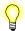# gf_context_set_transform()

Set the current 2D transform matrix

## Synopsis:

```#include <gf/gf.h>

void gf_context_set_transform(
gf_context_t context,
const gf_fixed_t *xform_matrix );```

## Arguments:

context
The draw context to set the transform for.
xform_matrix
A pointer to an array of four gf_fixed_t (signed 16.16 fixed point) elements composing the 2×2 matrix. Don't pass NULL for this argument.

gf

## Description:

This function sets the current 2D transform matrix. This 2×2 matrix is multiplied by all polygon, line, and polyline coordinates prior to rendering. Using a combination of the transform matrix and translations, various rotation, scaling and reflection operations are possible. See gf_context_set_translation() for information on translations.The 2D transform matrix applies only to geometrical primitives such as polygons, lines, and polylines. The algorithm isn't applied to spans, rectangles, bitmaps, fonts, etcetera.

The gf_fixed_t type is a 32-bit number representing signed 16.16 fixed point values used in transformation matrices. The composition of this type is native endian with the low 16 bits representing the fractional component, the high 16 bits representing the integral component, in two's complement notation.

## Classification:

QNX Graphics Framework

Safety:
Interrupt handler No
Signal handler No